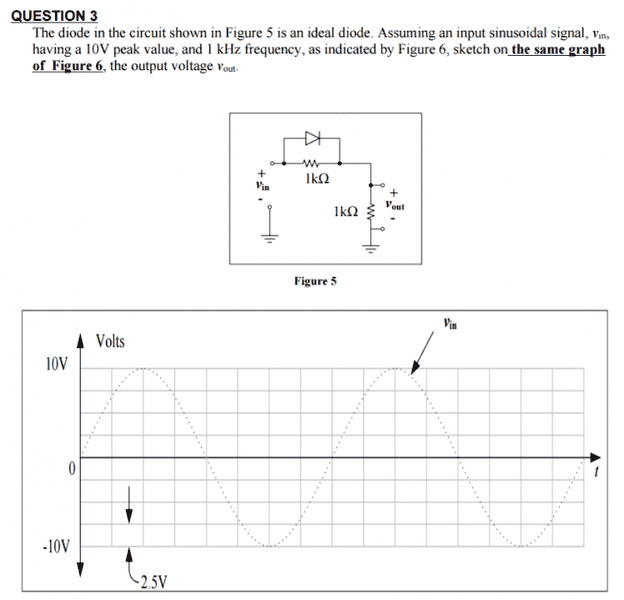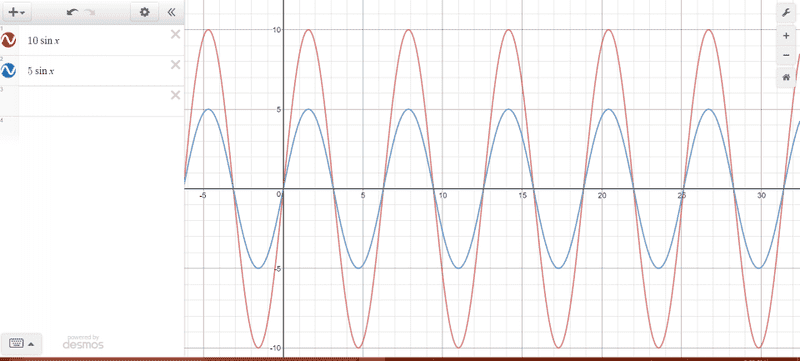Ideal diode question

Homework Statement

I am trying to prepare for an exam that is tomorrow, my professor gave sample questions but did not give us any solutions or answers. I have trouble with this question:The Attempt at a Solution

I don't know what to do. I would maybe try to figure out if the diode should be on or off. I would assume on in this case and then try to write vout as a function of vin? This isn't homework, I just really need to understand for my exam can someone please explain the steps to me ?

gneill
Mentor
Don't assume that the diode will always be on: the input is varying with time. Pick several locations along the input curve as test values and see what the circuit is doing for each. After one or two judiciously chosen points it should become clear how to sketch in the whole thing.

Don't assume that the diode will always be on: the input is varying with time. Pick several locations along the input curve as test values and see what the circuit is doing for each. After one or two judiciously chosen points it should become clear how to sketch in the whole thing.
What equation do I test those points in though ?

gneill
Mentor
What equation do I test those points in though ?
It's up to you to write equations for the circuit. You have two cases: diode conducting and diode not conducting. So two circuits to analyze.

It's up to you to write equations for the circuit. You have two cases: diode conducting and diode not conducting. So two circuits to analyze.
If it is on, then vin=vout? and the other case I don't know

It's up to you to write equations for the circuit. You have two cases: diode conducting and diode not conducting. So two circuits to analyze.
If I am going to have two equations, then which one am I going to draw ? Do I at least have the right idea, trying to express Vout as a function of Vin ? Or am I completely lost

gneill
Mentor
If it is on, then vin=vout?
Sure. For what parts of the input signal is it on?
and the other case I don't know
Why not? What is the path of the current in that case? What components does it flow through? What are the potential drops?
If I am going to have two equations, then which one am I going to draw ? Do I at least have the right idea, trying to express Vout as a function of Vin ? Or am I completely lost
Just pick a few individual values that the input can assume, like 0 V, 10 V, -10 V. See what the circuit is doing for each case. You'll be plotting points for BOTH versions of the circuit depending upon what the circuit is doing (diode conducting or not) at any particular time.

Sure. For what parts of the input signal is it on?

Why not? What is the path of the current in that case? What components does it flow through? What are the potential drops?

Just pick a few individual values that the input can assume, like 0 V, 10 V, -10 V. See what the circuit is doing for each case. You'll be plotting points for BOTH versions of the circuit depending upon what the circuit is doing (diode conducting or not) at any particular time.
Ok, so when it is off vout = vin/2 ?

gneill
Mentor
Ok, so when it is off vout = vin/2 ?
Yes, It's a simple voltage divider when the diode is off.

Sure. For what parts of the input signal is it on?

Why not? What is the path of the current in that case? What components does it flow through? What are the potential drops?

Just pick a few individual values that the input can assume, like 0 V, 10 V, -10 V. See what the circuit is doing for each case. You'll be plotting points for BOTH versions of the circuit depending upon what the circuit is doing (diode conducting or not) at any particular time.
Would it look something like this?Attachments

gneill
Mentor
No. That doesn't show the action of the diode. It looks like a "pure" voltage divider, affecting the whole input waveform equally.

Edit:
However, if you choose the appropriate portions of both curves using the conclusions you've drawn earlier, you should be able to piece together the entire output signal.

Last edited:
•Cocoleia